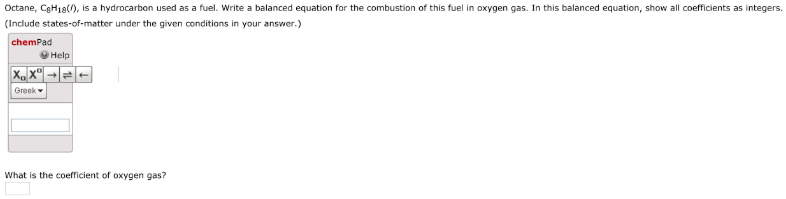# Octane, C8H18 (l), is a hydrocarbon used as a fuel. Write a balanced equation for the combustion of this fuel in oxygen gas. In this balanced equation, show all coefficients as integers. (Include states-of-matter under the given conditions in your answer.) What is the coefficient of oxygen gas?